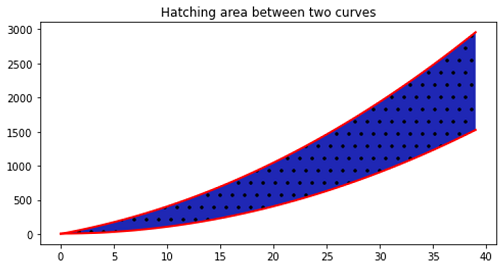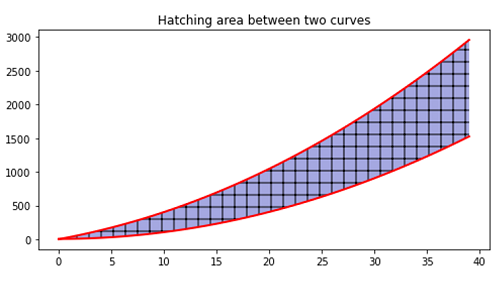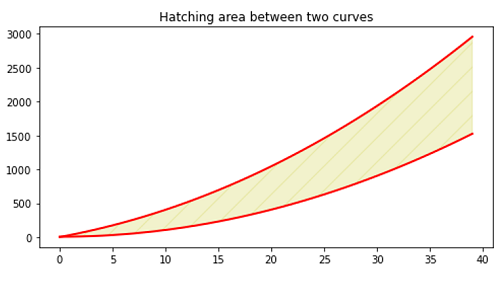# Python | Hatching the area between two curves

In this article, we are going to learn how to apply hatching for the area between two curves in x-y plot using matplotlib in Python?
Submitted by Anuj Singh, on September 01, 2020

Matplotlib has an inbuilt defined function for applying hatching to the area between two curves. Following example tries to do our desired operation with three different types of hatching.

```plt.text(0.45, 0.45, r'\$\biguplus\$')
```

Illustrations:## Python code for hatching the area between two curves

```import numpy as np
import matplotlib.pyplot as plt

#Definiing Curves
x = np.arange(40)
y = 4 + x**2
yy = x**2.05 + 29*x

#Hathing Area
plt.figure(figsize=(8,4))
plt.fill_between(x, y, yy, hatch='.')
plt.plot(x,y, linewidth=2.0, color='red')
plt.plot(x,yy, linewidth=2.0, color='red')
plt.title('Hatching area between two curves')

#Hathing Area
plt.figure(figsize=(8,4))
plt.fill_between(x, y, yy, alpha=0.4, hatch='+')
plt.plot(x,y, linewidth=2.0, color='red')
plt.plot(x,yy, linewidth=2.0, color='red')
plt.title('Hatching area between two curves')

#Hathing Area
plt.figure(figsize=(8,4))
plt.fill_between(x, y, yy, color='y', alpha=0.2, hatch='/')
plt.plot(x,y, linewidth=2.0, color='red')
plt.plot(x,yy, linewidth=2.0, color='red')
plt.title('Hatching area between two curves')
```

Output:

```Output is as Figure
```

Languages: » C » C++ » C++ STL » Java » Data Structure » C#.Net » Android » Kotlin » SQL
Web Technologies: » PHP » Python » JavaScript » CSS » Ajax » Node.js » Web programming/HTML
Solved programs: » C » C++ » DS » Java » C#
Aptitude que. & ans.: » C » C++ » Java » DBMS
Interview que. & ans.: » C » Embedded C » Java » SEO » HR
CS Subjects: » CS Basics » O.S. » Networks » DBMS » Embedded Systems » Cloud Computing
» Machine learning » CS Organizations » Linux » DOS
More: » Articles » Puzzles » News/Updates